#0
0
01. 云栖社区>
2. 博客>
3. 正文

## 机器学习利器——决策树和随机森林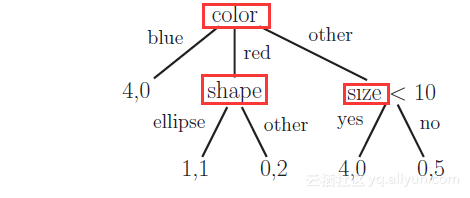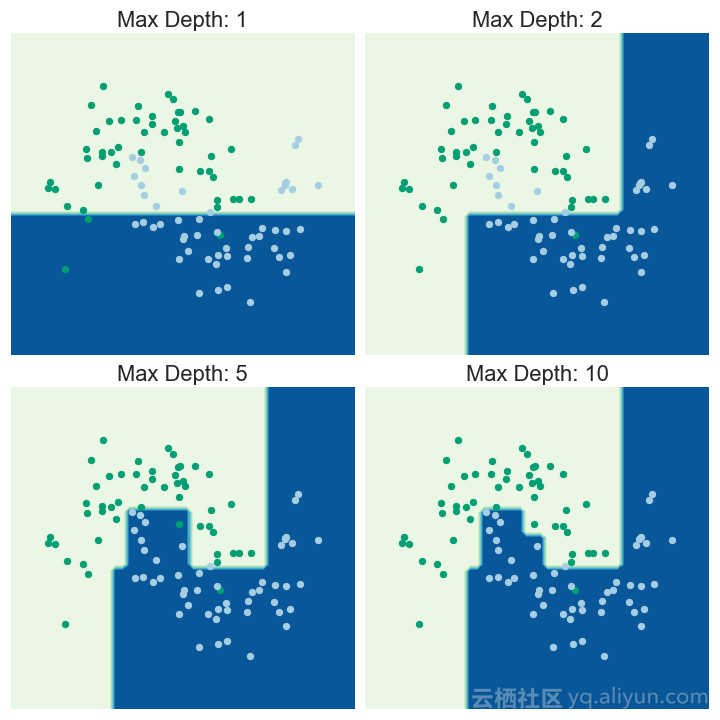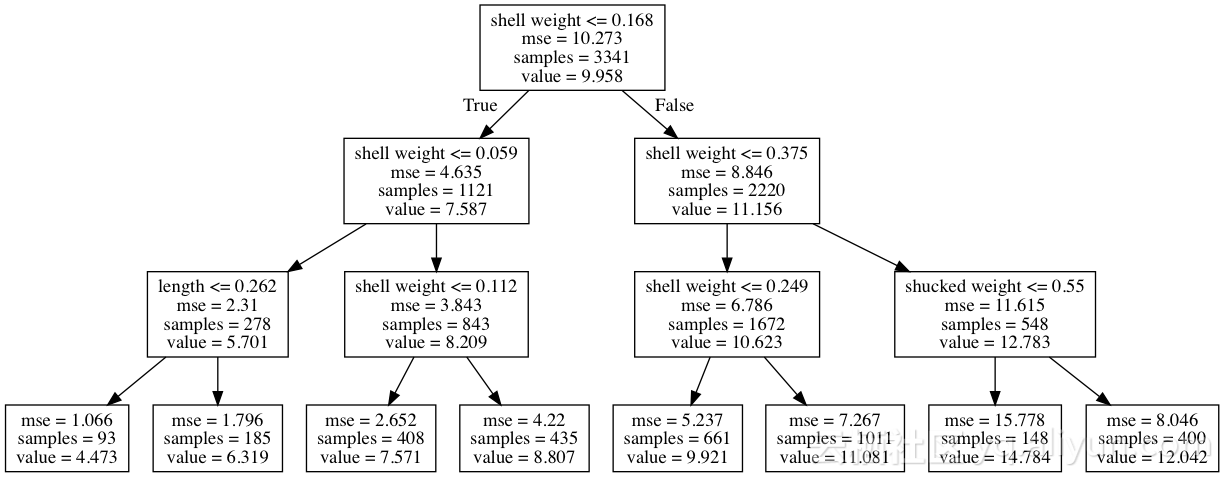(7.587 - 9.958) + (5.701 - 7.587) = -4.257

(4.473 - 5.701) = -1.228

``````from treeinterpreter import treeinterpreter as ti
dt_reg_pred, dt_reg_bias, dt_reg_contrib = ti.predict(dt_reg, X_test)
``````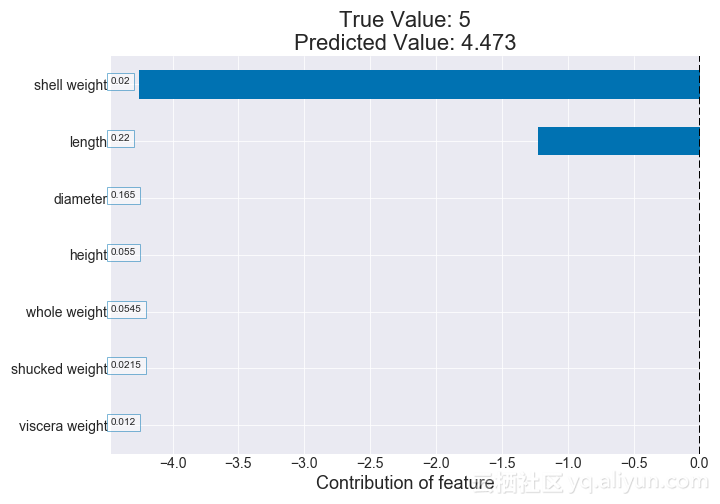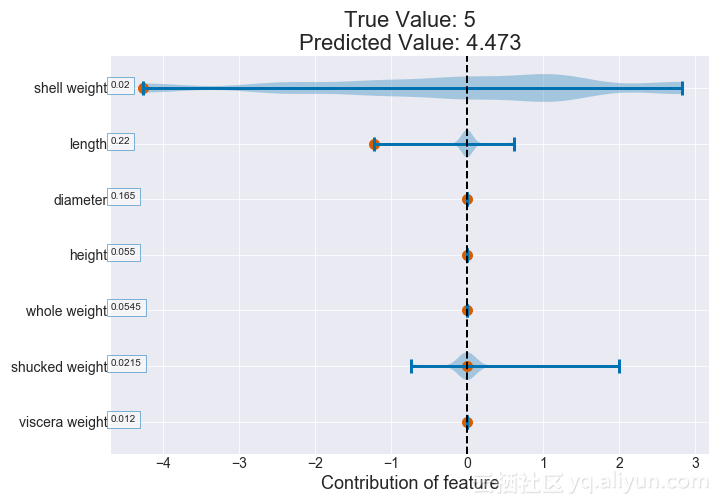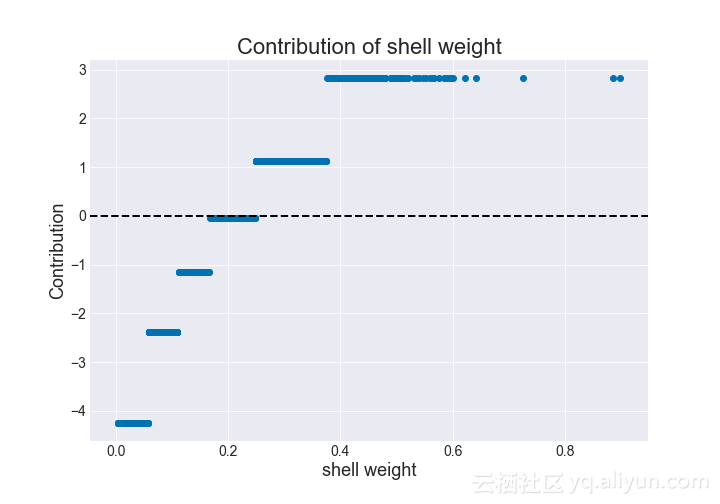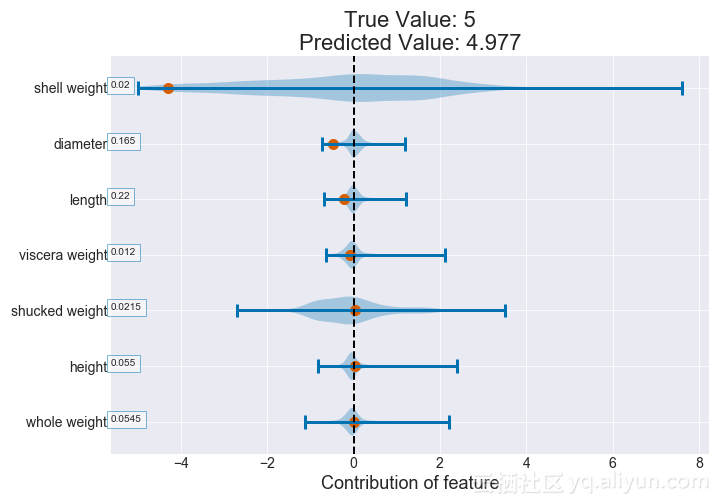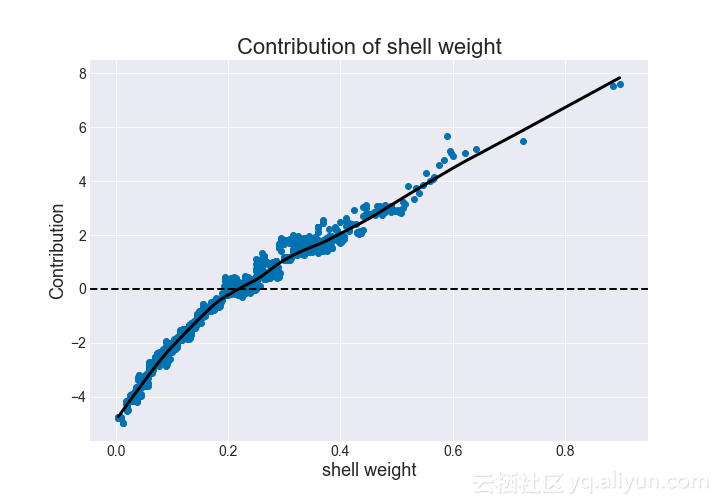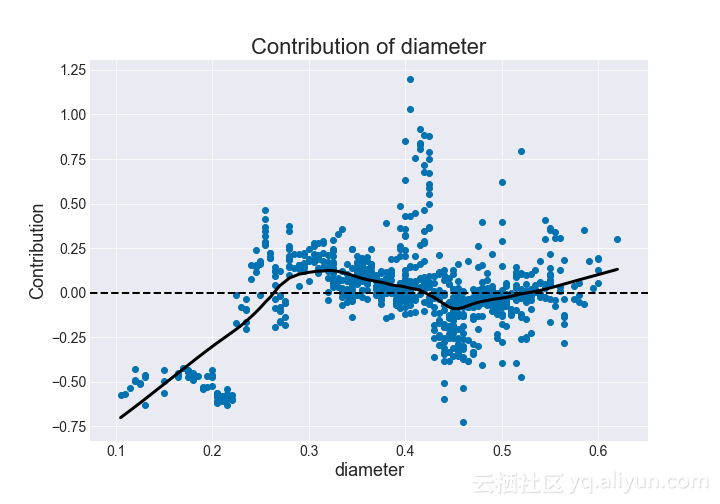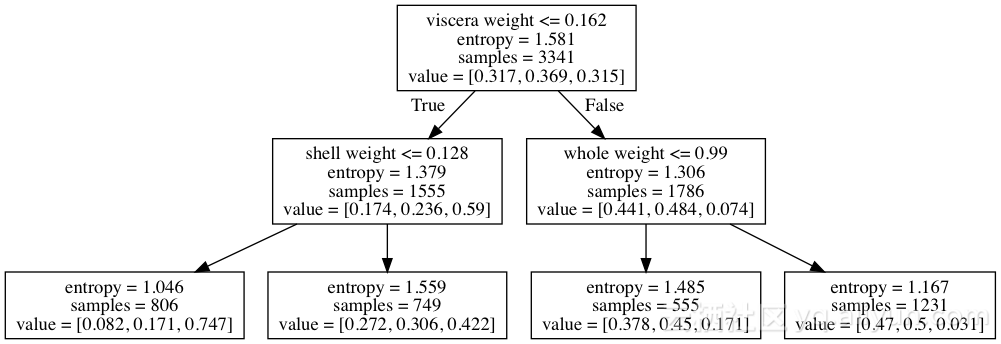(0.59 - 0.315) = 0.275

(0.747 - 0.59) = 0.157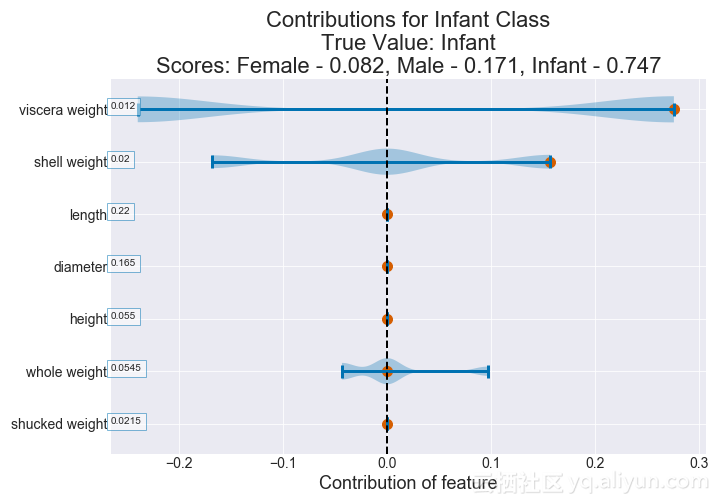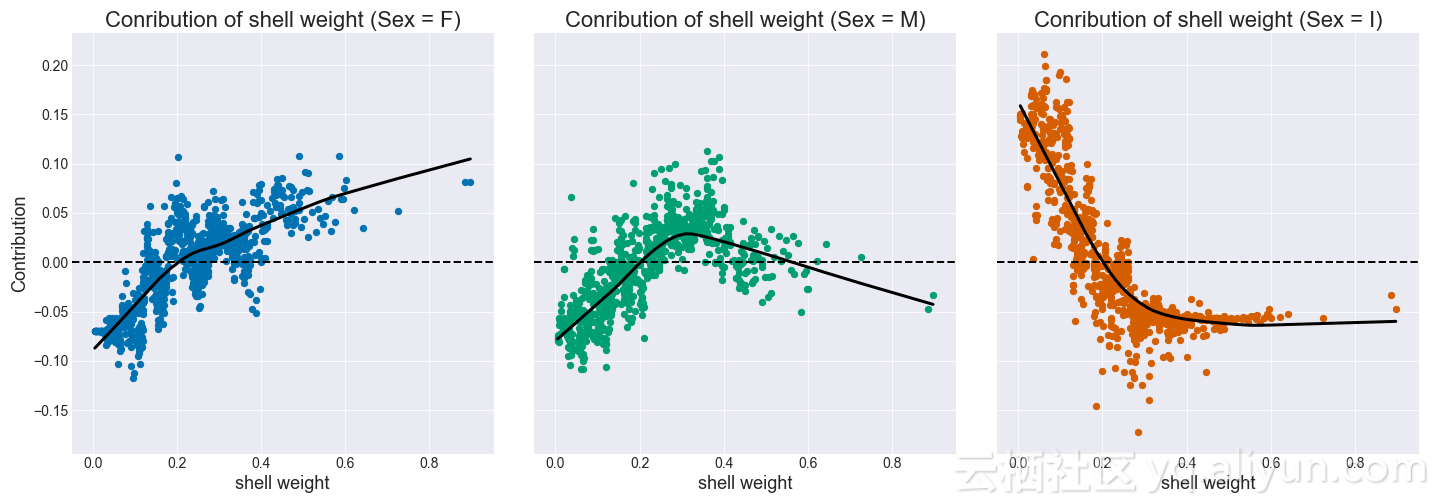+ 关注